# Math in Focus Grade 3 Chapter 7 Practice 4 Answer Key Multiplying Ones, Tens, and Hundreds with Regrouping

Go through the Math in Focus Grade 3 Workbook Answer Key Chapter 7 Practice 4 Multiplying Ones, Tens, and Hundreds with Regrouping to finish your assignments.

## Math in Focus Grade 3 Chapter 7 Practice 4 Answer Key Multiplying Ones, Tens, and Hundreds with Regrouping

Multiply and complete.

Question 1.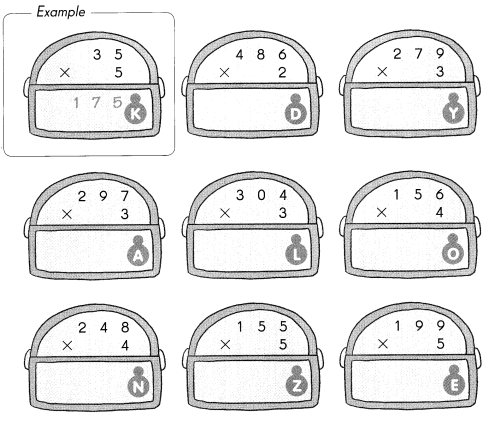What key cannot unlock treasure chests?

Write the letters which match the answers to find out.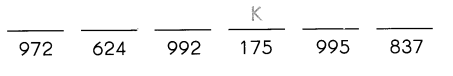The key for treasure chests is DONKEY.

Explanation:
The key for treasure chests is DONKEY.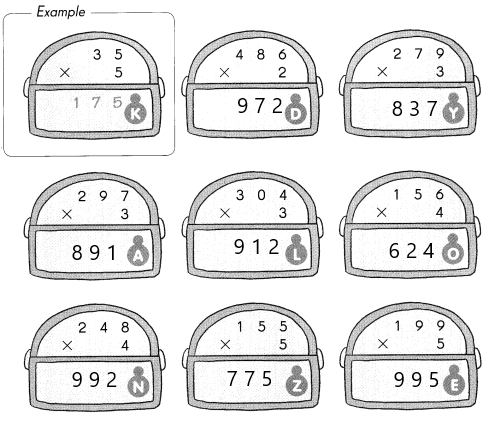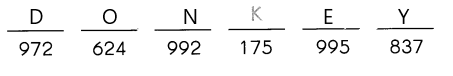Solve.

Example
Gina reads 84 pages of her book in a day. How many pages does Gina read in 5 days?
84 × 5 = 420
Gina reads 420 pages in 5 days.

Question 2.
187 cars are in a parking lot. Each car has 4 wheels. How many wheels do the cars have in all?
The number of wheels does the cars have in all is 748 wheels.

Explanation:
Given that 187 cars are in a parking lot and each car has 4 wheels. So the number of wheels does the cars have in all is 187×4 which is 748 wheels.

Question 3.
198 students attend a school. Each student carries 3 books. How many books do they carry in all?
The number of books does they carry in all is 594 books.

Explanation:
Given that 198 students attend a school and each student carries 3 books. So the number of books does they carry in all is 198×3 which is 594 books.

Question 4.
Jill feeds her pet hamster 5 food pellets each day. How many food pellets does she feed her hamster in 165 days?
Jill feeds 825 food pellets.

Explanation:
Given that Jill feeds her pet hamster 5 food pellets each day, so for 165 days Jill feeds 165×5 which is 825 food pellets.

Multiply.

Question 5.
450 × 2 = ______
450 × 2 = 900.

Explanation:
The multiplication of 450 and 2 is 900.

Question 6.
232 × 4 = ____
232 × 4 = 928.

Explanation:
The multiplication of 232 and 4 is 928.

Question 7.
259 × 3 = ______
259 × 3 = 777.

Explanation:
The multiplication of 259 and 3 is 777.

Question 8.
196 × 5 = ___
196 × 5 = 980.

Explanation:
The multiplication of 196 and 5 is 980.

Complete.

Question 9.
Circle the chest with the greatest product.
Here, we have circled the greatest product which is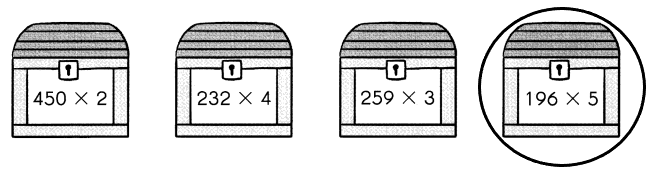Question 10.
Underline the chest with the least product.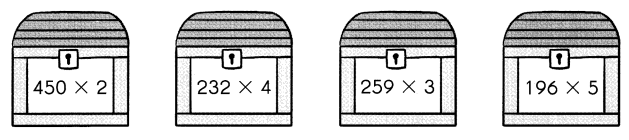Here, we have underlined the least product which is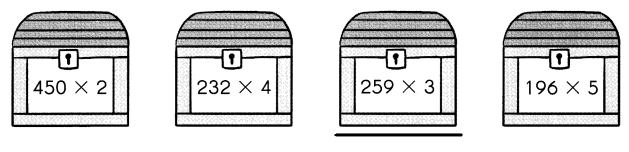Solve.
Then circle the chest with the correct answer.

Question 11.
Keith runs from Tree A to B to C, to D to E, then to F. The trees are planted 134 meters apart from each other. How for does Keith run in all?